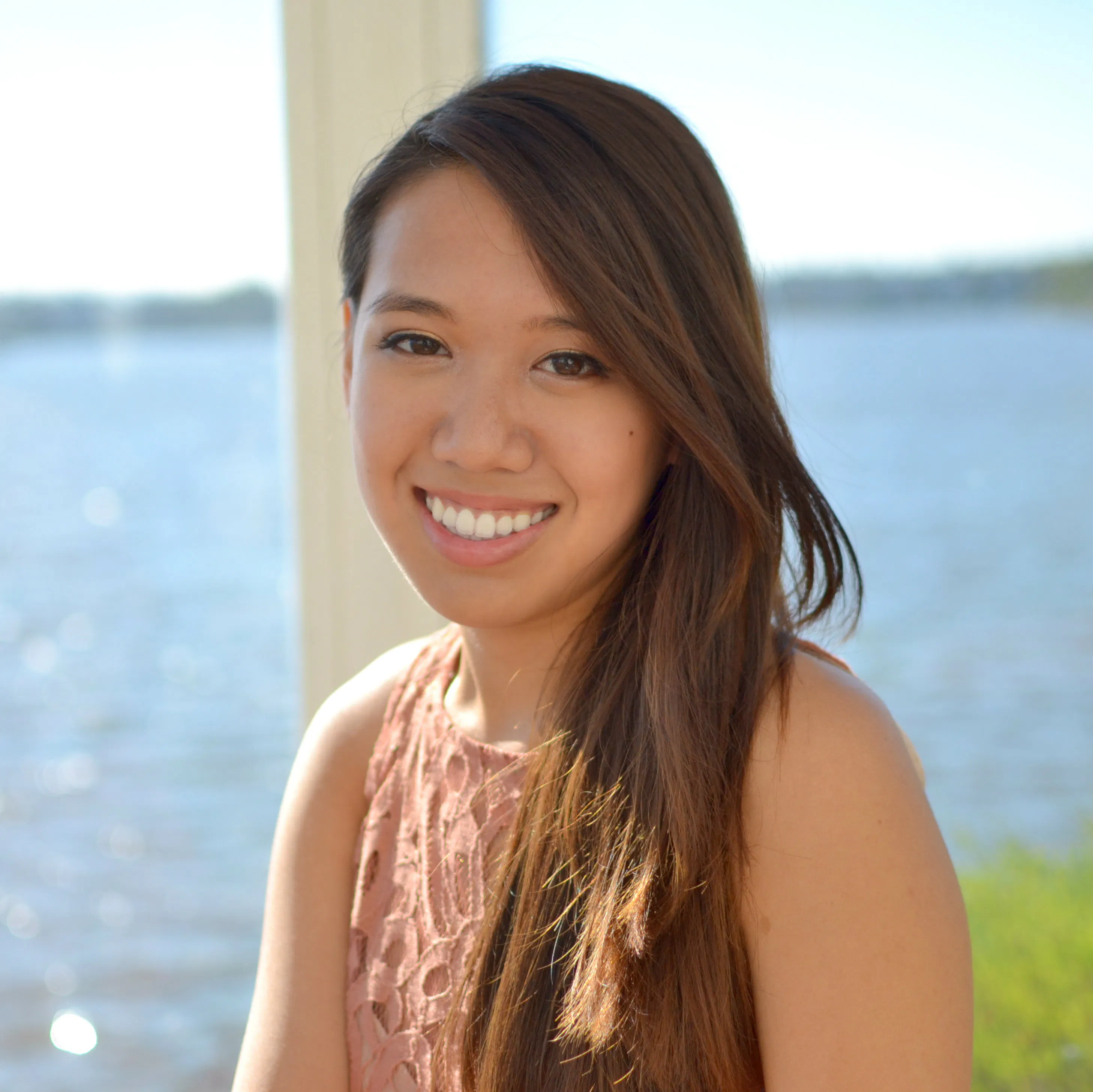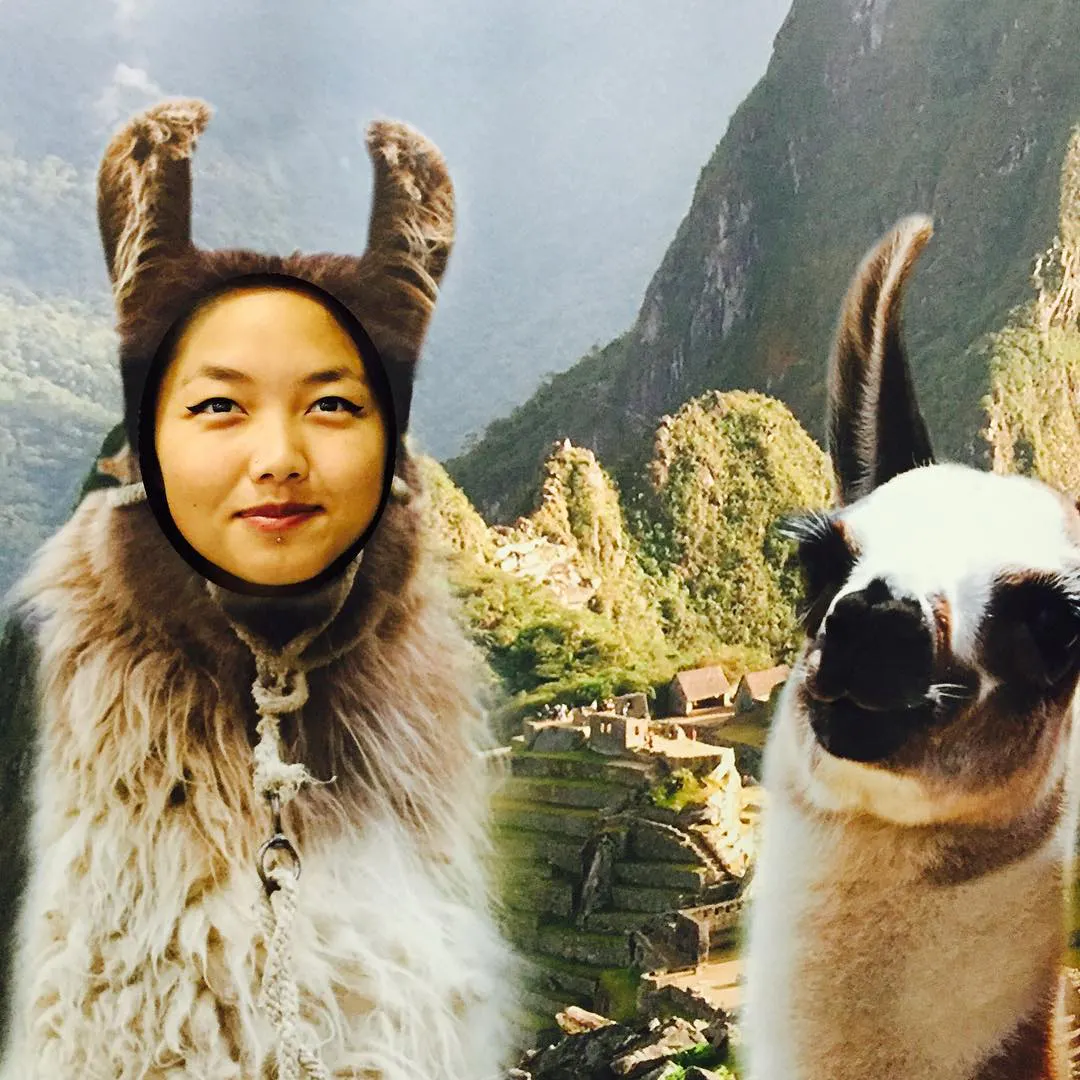Created December 9, 2015

# TrashScan

TrashScan is an interactive device that educates community members about sustainability in a fun and interactive way.

ExpertShowcase (no instructions)814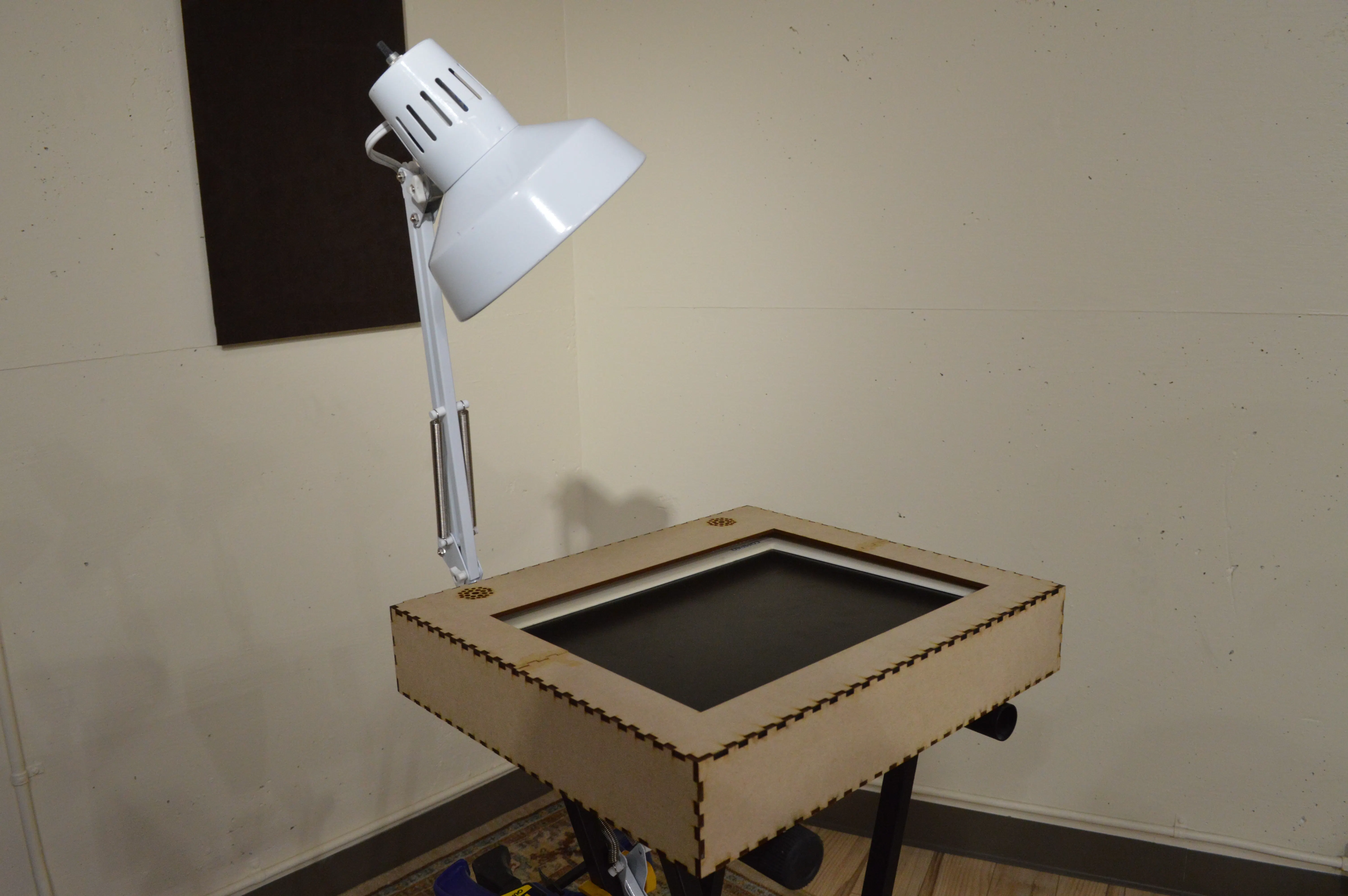## Things used in this project

### Hardware components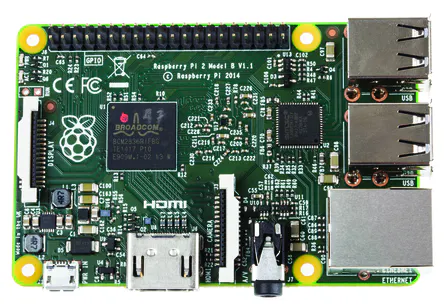Raspberry Pi 2 Model B
×1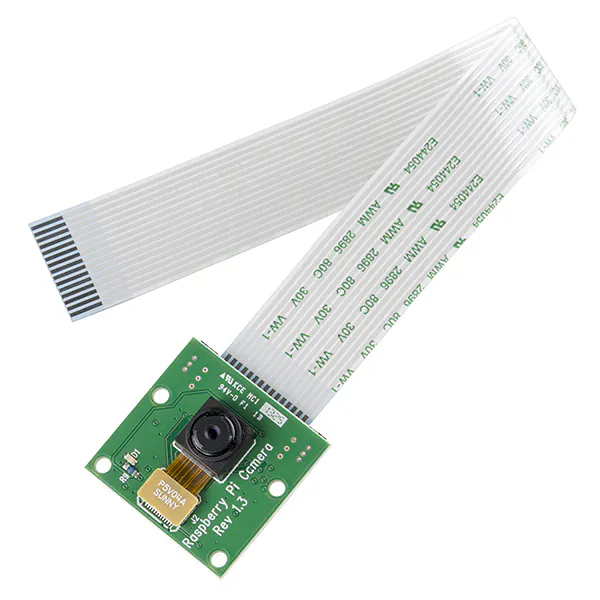Raspberry Pi Camera Module
×1
 Edimax USB 2.0 Wireless Adapter
×1
 Compaq FP745A LCD Computer Moniter
×1
 12"x24" Eco-Wood sheet
×3
 X-Frame Piano Stand
×1
 Lamp
×1
 Duct Tape
×1

### Software apps and online services

 CamFind This is the backend service which powered our image recognition.

### Hand tools and fabrication machines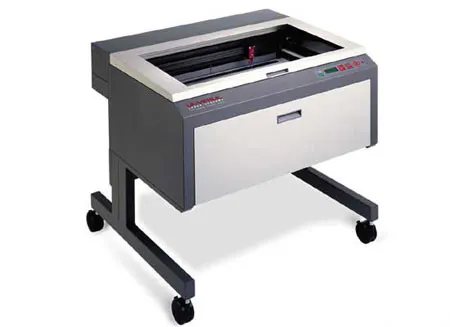Laser cutter (generic)3D Printer (generic)

## Code

### compost.pyde

Python
our animation for "Compost"
```import time

minim = Minim(this)
start = time.time()

# Half width of the shape.

# Speed of the shape.
XSpeed = 2.8
YSpeed = 2.2

# Starting position of shape.
xpos = 0
ypos = 0

# Left to Right.
xdirection = 1

# Top to Bottom.
ydirection = 1

dWidth = 1024
dHeight = 768

def setup():
size(dWidth, dHeight)
f = createFont("Georgia", 30)
textFont(f)
player.play()
global comp1_cycle
comp1_cycle = loadImage("compost1_cycle.png")    # Load the image into the program
global comp2_text
global comp3_spoons
global comp4

global xpos, ypos
noStroke()
frameRate(30)
# Set the starting position of the shape.
xpos = width / 2
ypos = height / 2

def draw():
dWidth = 1024
dHeight = 768
# image(img,0,0)
background(255)
targetX = dWidth/3.5
targetY = dHeight/2
# TARGET

#first outer circle
stroke(255,0,0)
strokeWeight(60)
noFill()
ellipseMode(CENTER)

# big red center
fill(255,0,0)
ellipseMode(CENTER)

#2nd outer circle
stroke(255,0,0)
strokeWeight(50)
noFill()
ellipseMode(CENTER)

# image(img, displayWidth-400, displayHeight / 3, img.width / 4, img.height / 4)
# rotatePaper()
#tint(255, 165)
image(comp1_cycle, (dWidth-(dWidth/3))+50, dHeight/35, width/6, height/4)
image(comp2_text, dWidth-400, (dHeight/3)-100, width/3, height/3)
image(comp3_spoons, (dWidth)/2+(dWidth)/10, (dHeight-(dHeight/3)), dWidth/7, dHeight/7)
# image(comp5_foods, (displayWidth)/2+(displayWidth)/4+30, (displayHeight-(displayHeight/3)),displayWidth/5, displayHeight/5)
textSize(100)
fill(0, 102, 153, 204)
originX = dWidth-600
#text("COMPOST", (displayWidth)/2+(displayWidth)/7, (displayHeight/2), -30)  # Specify a z-axis value
textSize(22)
fill(random(256), random(256), random(256))
text("If it was alive before, you can compost!", dWidth-400, (dHeight-(dHeight/3))-50)

global xpos, ypos, xdirection, ydirection
# Update the position of the shape.
xpos += 1.5*XSpeed * xdirection
ypos += 1.5*YSpeed * ydirection

# Test to see if the shape exceeds the boundaries of the screen.
# If it does, reverse its direction by multiplying by -1.
if (xpos < Radius) or (width - Radius < xpos):
xdirection *= -1

if (ypos < Radius) or (height - Radius < ypos):
ydirection *= -1

saveFrame()

end = time.time()
if end-start > 10:
exit()

```

### default.pyde

Python
our default view including instructions
```dWidth = 1024
dHeight = 768

def setup():
# dWidth = 1024
# global dHeight = 768
size(dWidth, dHeight)
# size(1440,266)
# noLoop()
# time.sleep(10)
# exit()

def draw():
dWidth = 1024
dHeight = 768
# image(img,0,0)
background(255)
targetX = dWidth/3.5
targetY = dHeight/2
# TARGET

#first outer circle
stroke(255,0,0)
strokeWeight(60)
noFill()
ellipseMode(CENTER)

# big red center
fill(255,0,0)
ellipseMode(CENTER)

#2nd outer circle
stroke(255,0,0)
strokeWeight(50)
noFill()
ellipseMode(CENTER)

textSize(20)
font = createFont("Gotham", 50)
textFont(font)
fill(0, 102, 153, 204)
textY = dHeight / 3
textX = dWidth-420
text("INSTRUCTIONS", textX, textY)  # Specify a z-axis value
textSize(20)
text("1) Place trash on the red target.", textX, textY+50)
text("2) Wait for camera to capture trash.", textX, textY+100)
text("3) See what TrashScan says!", textX, textY+150)
textY = dHeight / 3
textX = dWidth-390
beginMessage = "LET'S START!"
textSize(48)
fill(random(256), random(256), random(256))
text(beginMessage, textX, textY + 300)

saveFrame()

```

### landfill.pyde

Python
animation for "Landfill"
```import time
minim = Minim(this)
start = time.time()

# Half width of the shape.

# Speed of the shape.
XSpeed = 2.8
YSpeed = 2.2

# Starting position of shape.
xpos = 0
ypos = 0

# Left to Right.
xdirection = 1

# Top to Bottom.
ydirection = 1

dWidth = 1024
dHeight = 768

def setup():
size(dWidth, dHeight)
#mp3
player.play()

player.play()
#text
#printArray(PFont.list())
f = createFont("Gotham", 46)
textFont(f)
#image
global img
img = loadImage("landfill.jpg")    # Load the image into the program
global bin_img
global trash1
global trash2
global trash3
global trash4
# noLoop()
global xpos, ypos
noStroke()
frameRate(30)
# Set the starting position of the shape.
xpos = width / 2
ypos = height / 2

def draw():
dWidth = 1024
dHeight = 768
# image(img,0,0)
background(255)
targetX = dWidth/3.5
targetY = dHeight/2
# TARGET

#first outer circle
stroke(255,0,0)
strokeWeight(60)
noFill()
ellipseMode(CENTER)

# big red center
fill(255,0,0)
ellipseMode(CENTER)

#2nd outer circle
stroke(255,0,0)
strokeWeight(50)
noFill()
ellipseMode(CENTER)

#IMAGE STUFF
tint(255, 165)
image(img, dWidth-450, dHeight/4, width/2, height/2)
#textAlign(LEFT)
#drawType(0.75)
noTint()
fill(0,0,0)
textSize(75)
text("LANDFILL", dWidth-400, (dHeight/2))
textSize(14)
text("The average American produces 102 tons of trash in their lifetime.", (dWidth)/3+(dWidth)/5, (dHeight/5))

global xpos, ypos, xdirection, ydirection
# Update the position of the shape.
xpos += 1.5*XSpeed * xdirection
ypos += 1.5*YSpeed * ydirection

# Test to see if the shape exceeds the boundaries of the screen.
# If it does, reverse its direction by multiplying by -1.
if (xpos < Radius) or (width - Radius < xpos):
xdirection *= -1

if (ypos < Radius) or (height - Radius < ypos):
ydirection *= -1

# Draw the shape

saveFrame()

end = time.time()
if end-start > 15:
exit()

```

### mixedpaper.pyde

Python
animation for "Mixed Paper"
```import time
start = time.time()

minim = Minim(this)

# Half width of the shape.
# Radius2 = 30

# Speed of the shape.
XSpeed = 2.8
YSpeed = 2.2

# Starting position of shape.
xpos = 0
ypos = 0

# Left to Right.
xdirection = 1

# Top to Bottom.
ydirection = 1

def setup():
size(displayWidth, displayHeight)
# size(1440,266)
global img
global yellowpaper
global postits
# noLoop()

player.cue(77500)
player.play()

global xpos, ypos
noStroke()
frameRate(30)
# Set the starting position of the shape.
xpos = width / 2
ypos = height / 2

def draw():
# image(img,0,0)
background(255)
targetX = displayWidth/3
targetY = displayHeight/2
# TARGET

#first outer circle
stroke(255,0,0)
strokeWeight(60)
noFill()
ellipseMode(CENTER)

# big red center
fill(255,0,0)
ellipseMode(CENTER)

#2nd outer circle
stroke(255,0,0)
strokeWeight(60)
noFill()
ellipseMode(CENTER)

global xpos, ypos, xdirection, ydirection
# Update the position of the shape.
xpos += 1.5*XSpeed * xdirection
ypos += 1.5*YSpeed * ydirection

# Test to see if the shape exceeds the boundaries of the screen.
# If it does, reverse its direction by multiplying by -1.
if (xpos < Radius) or (width - Radius < xpos):
xdirection *= -1

if (ypos < Radius) or (height - Radius < ypos):
ydirection *= -1

# Draw the shape

# YELLOW PAPER
global xpos, ypos
# Update the position of the shape.
xpos += 2*XSpeed * xdirection
ypos += 2*YSpeed * ydirection

# Test to see if the shape exceeds the boundaries of the screen.
# If it does, reverse its direction by multiplying by -1.
# if (xpos < Radius) or (width - Radius < xpos):
#     xdirection *= -1

if (ypos < Radius) or (height - Radius < ypos):
ydirection *= -1

# Draw the shape

# POST ITS
global xpos, ypos
# Update the position of the shape.
xpos += 1*XSpeed * xdirection
ypos += 1*YSpeed * ydirection

# Test to see if the shape exceeds the boundaries of the screen.
# If it does, reverse its direction by multiplying by -1.
# if (xpos < Radius) or (width - Radius < xpos):
#     xdirection *= -1

if (ypos < Radius) or (height - Radius < ypos):
ydirection *= -1

# Draw the shape

font = createFont("Gotham", 70)
fill(41, 64, 255, 200)
# text("MIXED PAPER", displayWidth-500, 200, -40)  # Specify a z-axis value
fill(41, 64, 255, 240)
text("MIXED PAPER", displayWidth-499, 210)  # Default depth, no z-value specified

# textSize(36)
font = createFont("Gotham", 36)
textFont(font)
# fill(0, 102, 153, 204)
textY = displayHeight / 3
textX = displayWidth-540
text("Do you know the 3 R's?", textX, textY)  # Specify a z-axis value
textSize(60)
text("REDUCE", textX, textY+100)
text("REUSE", textX, textY+200)
text("RECYCLE", textX, textY+300)

saveFrame()

end = time.time()
if end - start >= 10:
exit()
```

### bottles_cans.pyde

Python
animation for "Bottles & Cans"
```import time
minim = Minim(this)
start = time.time()

# Half width of the shape.

# Speed of the shape.
XSpeed = 2.8
YSpeed = 2.2

# Starting position of shape.
xpos = 0
ypos = 0

# Left to Right.
xdirection = 1

# Top to Bottom.
ydirection = 1

dWidth = 1024
dHeight = 768

def setup():
size(dWidth, dHeight)
#mp3
player.play()
#text
# printArray(PFont.list())
f = createFont("Georgia", 30)
textFont(f)
#image
global img
img = loadImage("cans.png")    # Load the image into the program
global bin_img
global can_img
global can3_img
global can4_img
global can5_img
# noLoop()
global xpos, ypos
noStroke()
frameRate(30)
# Set the starting position of the shape.
xpos = width / 2
ypos = height / 2

def draw():
dWidth = 1024
dHeight = 768
# image(img,0,0)
background(255)
targetX = dWidth/3.5
targetY = dHeight/2
# TARGET

#first outer circle
stroke(255,0,0)
strokeWeight(60)
noFill()
ellipseMode(CENTER)

# big red center
fill(255,0,0)
ellipseMode(CENTER)

#2nd outer circle
stroke(255,0,0)
strokeWeight(50)
noFill()
ellipseMode(CENTER)

#image(bin_img, (displayWidth)/2+(displayWidth)/8, 0, displayHeight/5, displayHeight/3)
tint(255, 140)
image(img, dWidth-400, dHeight/3, dWidth/3, dHeight/4)
#textAlign(LEFT)
#drawType(0.75)
noTint()
fill(0,0,0)
textSize(48)
text("BOTTLES & CANS", dWidth-430, (dHeight/2))

global xpos, ypos, xdirection, ydirection
# Update the position of the shape.
xpos += 1.5*XSpeed * xdirection
ypos += 1.5*YSpeed * ydirection

# Test to see if the shape exceeds the boundaries of the screen.
# If it does, reverse its direction by multiplying by -1.
if (xpos < Radius) or (width - Radius < xpos):
xdirection *= -1

if (ypos < Radius) or (height - Radius < ypos):
ydirection *= -1

# Draw the shape

saveFrame()

end = time.time()
if end-start > 10:
exit()

```

### TrashScan

Here is the python source code linked on github.

## Credits

### Rachel Lin

2 projects • 0 followers
Designer + Developer

### Jessie Salas

3 projects • 1 follower
Machine learning and NLP

### Drake Myers

5 projects • 3 followers